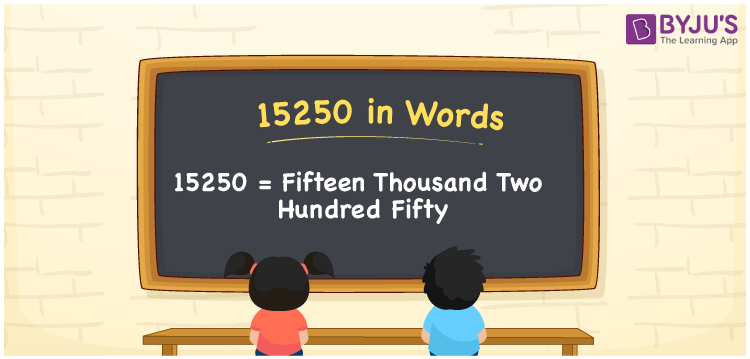# 15250 in Words

We can write 15250 in words as Fifteen Thousand Two Hundred Fifty. In other words, we can say that the number name of 15250 is Fifteen Thousand Two Hundred Fifty. As we know, number names are used to express a numerical value. Suppose you spotted a price, say Rs. 15, 250, on a banner; you may spell it as Fifteen thousand two hundred fifty rupees. Let’s learn how to derive the word form of 15250 in an easily understandable method here in this article.

 15250 in words Fifteen Thousand Two Hundred Fifty Fifteen thousand two hundred fifty in Numbers 15250

## 15250 in English Words

Generally, we use the English alphabet to write numbers in words. Therefore, we can express 15250 in English words as “Fifteen Thousand Two Hundred Fifty”.## How to Write 15250 in Words?

The number 15250 has five digits. So, we need to make a place value chart with five columns, as shown below, to convert the number 15250 into words.

 Ten thousand Thousands Hundreds Tens Ones 1 5 2 5 0

Here, ones = 0, tens = 5, hundreds = 2, thousands = 5, ten thousand = 1

These digits can be expanded as follows.

1 × Ten thousand + 5 × Thousand + 2 × Hundred + 5 × Ten + 0 × One

= 1 × 10000 + 5 × 1000 + 2 × 100 + 5 × 10 + 0 × 1

= 10000 + 5000 + 200 + 50

= Ten Thousand + Five thousand + Two hundred + Fifty

= Fifteen thousand + Two hundred fifty

= Fifteen Thousand Two Hundred Fifty

15250 is a natural number that is the successor of 15249 and the predecessor of 15251.

15250 in words – Fifteen thousand two hundred fifty

Is 15250 an odd number? – No

Is 15250 an even number? – Yes

Is 15250 a prime number? – No

Is 15250 a composite number? – Yes

Is 15250 a perfect square number? – No

Is 15250 a perfect cube number? – No

## Frequently Asked Questions on 15250 in Words

Q1

### How do you write 15250 in words?

We can write 15250 in English words as Fifteen thousand two hundred fifty.
Q2

### What number is Fifteen thousand two hundred fifty in words?

The number 15250 is expressed as Fifteen thousand two hundred fifty in words.
Q3

### Find 15250 + 3840. Express the answer in words.

15250 + 3840 = 19090 Therefore, the value of 15250 + 3840, i.e., 19090 in words is Nineteen thousand and ninety.
Q4

### What is the place value of 2 in the number 15250?

The place value of 2 in the number 15250 is 2 х 100 = 200
Q5

### What is the face value of 2 in the number 15250?

The face value of 2 in the number 15250 is 2 only.